From: Meshaal Abdulla, a student

Find an expression for 1-3+5 - 7 + 9 - 11 + ... + (-1)^(n-1) * (2n-1) and PROVE that it is correct.

Hi Meshaal.

We start by figuring out an expression that we think will work, then we later prove it works. So let's make a table of values using the smaller values of n to see if there is a pattern. Let's call the nth term an.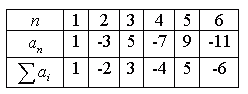You can immediately see patterns here. It looks like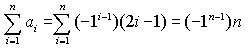So that's our hypothesis. We've checked a few small values of n and so we know that this works for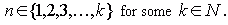In particular, we know that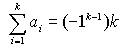So we can prove the general case for n if we can show that the case for k implies the case for k+1.

We can begin this proof by writing out an expansion of the k+1 case: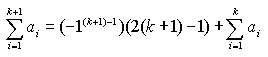So we've expanded out the largest term and left the rest in sigma notation. Notice that this is exactly what we earlier said we know! So we can substitute that value in for the sigma notation: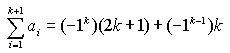Now it is left to you to simplify this (start by factoring the -1 exponent). You should get the general case for k+1, which means it is true for n (by induction).

Hope this helps!
Stephen La Rocque.